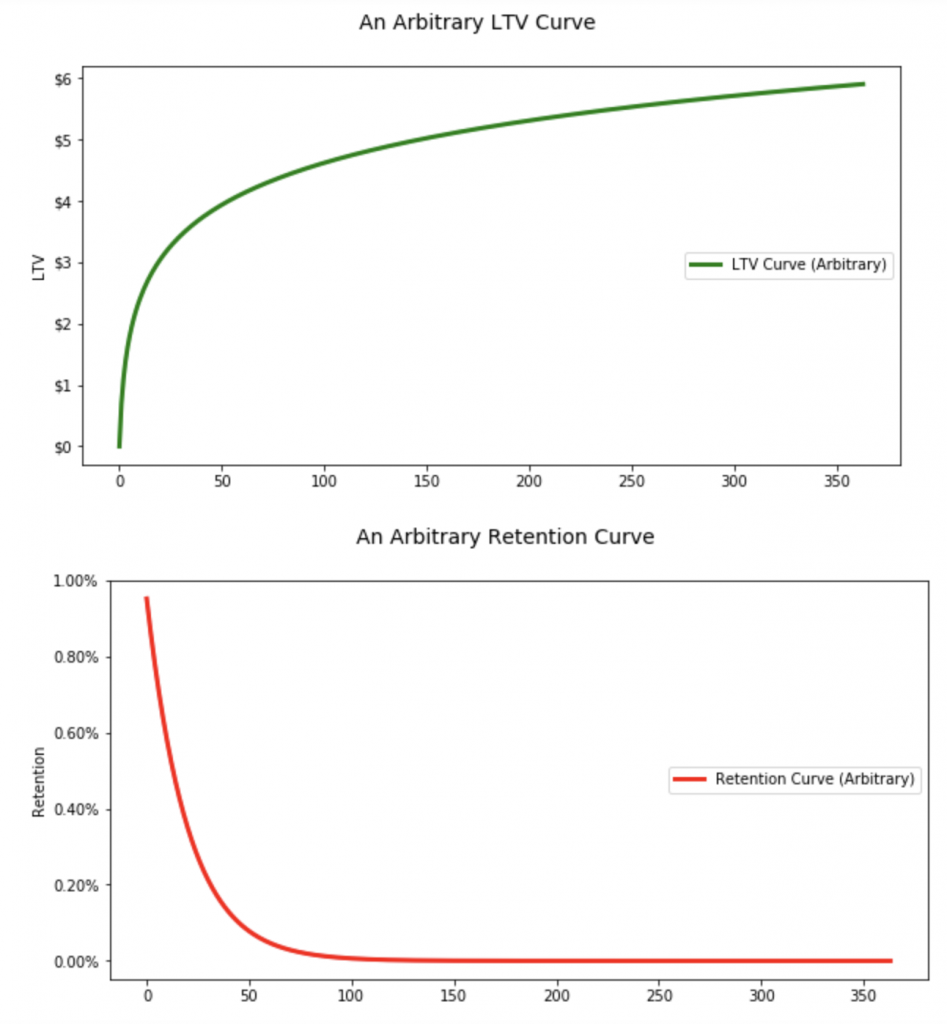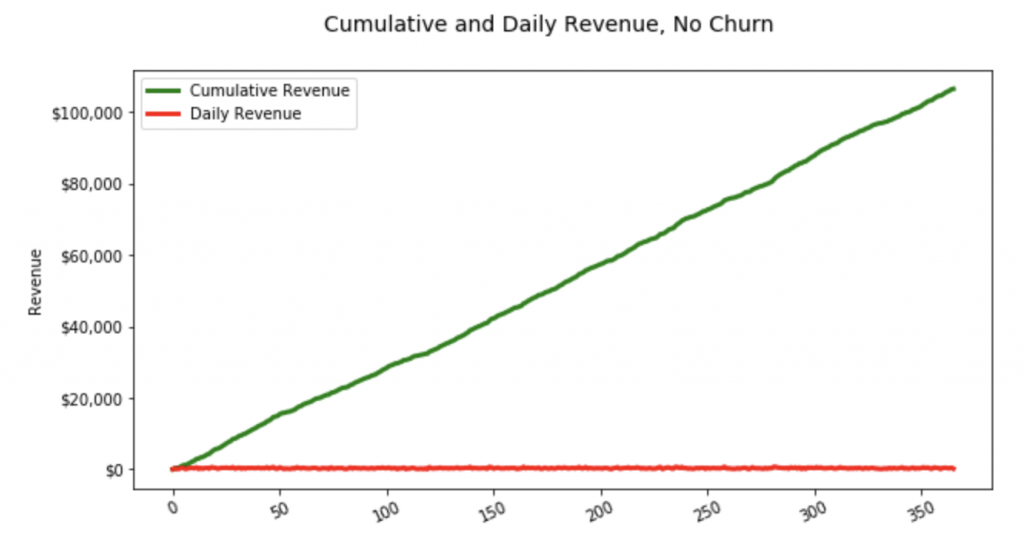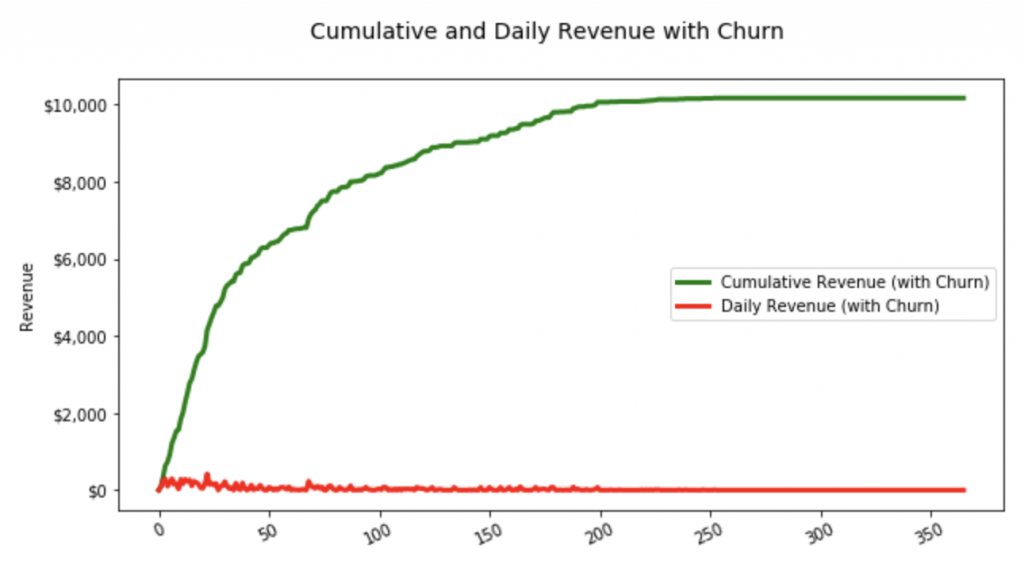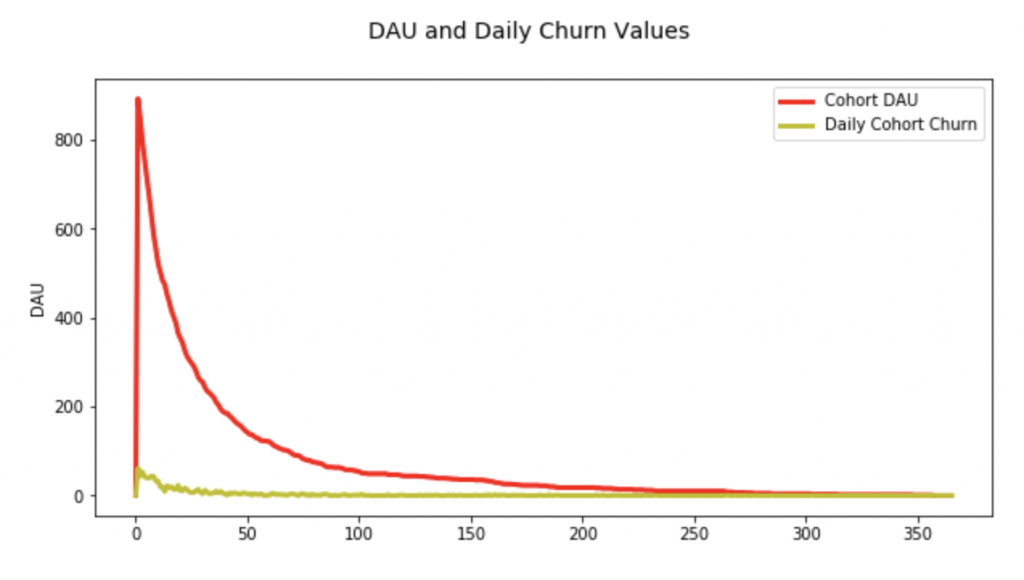# Visualizing the dynamic between LTV and Retention## Showcasing how product retention impacts overall product LTV.

One common mistake made in freemium product analysis is the conceptual unbundling of the LTV curve from the Retention curve. When these curves are presented in the abstract (for instance, in an article about how to calculate LTV), they often look, superficially, of a similar shape, almost as if they are the inverse of each other. With a quick glance, it’s understandable that one might assume that LTV and Retention are really just independent measurements of the same phenomenon. Below are completely arbitrarily constructed sample LTV and Retention curves:The fact of the matter is that LTV is completely dependent on Retention — it is calculated and projected on the basis of user retention, and any LTV calculation needs to be utilized with that in mind. What gives most freemium LTV curves the distinctive “bowed” shape (and why most LTV estimates are calculated with either logarithmic or exponential formulas) is retention: since LTV estimates are cohort-based (ie. what a cohort is expected to be worth at some point in the future), they are necessarily impacted by cohort retention: the LTV curve inflects downward because members of a cohort can’t spend money if they have churned out of the product.

It’s easy to conceptualize this by demonstrating what happens to a cohort that experiences no churn; every user in a cohort stays within the product every day for a year. This simple simulation can be done by creating a 1,000-person user base with some characteristics:

• Any given user has a 5% chance of being a “payer”;
• On any given day, payers have some probability of making a randomly-determined payment;
• On any given day, all users have some random but constant probability between 1% and 10% of churning (ie. their churn probability is the same every day but is randomly determined when they join the product).

The Python code to create such a user base looks like this:

```import matplotlib.pyplot as plt
import matplotlib.ticker as ticker
import pandas as pd
import numpy as np
import random

def build_userbase( n, payer_percentage ):
users = pd.DataFrame( columns=[ "user", "payer", "payment_probability", "payment" ] )
for x in range( 1, n + 1 ):
payer = True if random.randint( 1, 100 ) <= ( payer_percentage * 100 ) else 0
payment_probability = 0
payment = 0
churn_probability = float( random.randint( 1, 10 ) ) / 100
if payer:
payment_probability = float( random.randint( 1, 25 ) ) / 100
payment = float( random.randint( 1, 100 ) )
users = users.append(
{ "user": x, "payer": payer,
"payment_probability": payment_probability, "payment": payment,
"churn_probability": churn_probability, "churned": 0 }, ignore_index=True )

return users

#
# Build initial userbase
#

users = build_userbase( 1000, payer_percentage=0.05 )
users[ "churned" ] = users[ "churned" ].astype('bool')```

With the user base generated, daily revenue values without churn — meaning, each user’s churn probability is ignored and each of the 1,000 users is present in the product every day, with payers paying on any given day based on their payment probability — are produced and plotted for a period of one year with the following code:

```def build_cumulative_revenue( users, days ):
payers = users[ users[ 'payer' ] == 1 ]
daily_revenue = [ 0 ] * ( days + 1 )
daily_cumulative_revenue = [ 0 ] * ( days + 1 )
for x in range( 1, days + 1 ):
daily_revenue[ x ] = 0
daily_cumulative_revenue[ x ] = 0
this_daily_revenue = 0
for index, p in payers.iterrows():
this_payment_probability = float( random.randint( 1, 100 ) ) / 100
this_payment = p[ "payment" ] if this_payment_probability <= p[ "payment_probability" ] else 0
this_daily_revenue += this_payment

daily_revenue[ x ] = this_daily_revenue
daily_cumulative_revenue[ x ] = ( daily_cumulative_revenue[ x - 1 ] + daily_revenue[ x ] ) if x > 1 else daily_revenue[ x ]

return daily_revenue, daily_cumulative_revenue

#
# Get daily revenue values
#

dr_users = users
daily_revenue, daily_cumulative_revenue = build_cumulative_revenue( dr_users, 365 )

#
# Print Revenue Graph
#

fig, ax = plt.subplots()
plt.rcParams['figure.figsize'] = [10, 5]
plt.plot( daily_cumulative_revenue, '-g', label='Cumulative Revenue', linewidth=3 )
plt.plot( daily_revenue, '-r', label='Daily Revenue', linewidth=3 )
plt.legend(loc='upper left')
plt.ylabel( 'Revenue' )
fmt = '\${x:,.0f}'
tick = ticker.StrMethodFormatter( fmt )
ax.yaxis.set_major_formatter( tick )
plt.xticks( rotation=25 )
fig.suptitle( 'Cumulative and Daily Revenue, No Churn', fontsize=14 )
plt.show()```

With the resultant graph looking like this: a straight line that goes up and to the right (the red line is daily revenue generated and the green line is cumulative revenue over the period):This is what one would expect to see if users never left a product — the payers continue to contribute revenue to the app and cumulative revenue never “bows” down.

Adding churn into the calculation changes the picture. The following code produces daily revenue and cumulative revenue values over the course of a year but takes into account each user’s pre-determined probability of churning — that is, on each day, the user has a possibility of churning out of the product and never returning. This is done with the following code:

```def build_cumulative_revenue_with_churn( users, days ):
payers = users[ users[ 'payer' ] == 1 ]
daily_revenue = [ 0 ] * ( days + 1 )
daily_cumulative_revenue = [ 0 ] * ( days + 1 )
for x in range( 1, days + 1 ):
daily_revenue[ x ] = 0
daily_cumulative_revenue[ x ] = 0
this_daily_revenue = 0
for index, p in payers.iterrows():
if( not p[ "churned" ] ):
#if they didn't churn out
this_churn_probability = float( random.randint( 1, 100 ) ) / 100
if this_churn_probability > p[ "churn_probability" ]:
#if this isn't their day to churn
this_payment_probability = float( random.randint( 1, 100 ) ) / 100
this_payment = p[ "payment" ] if this_payment_probability <= p[ "payment_probability" ] else 0
this_daily_revenue += this_payment
else:
#they are churning
payers.loc[ index, "churned" ] = True

daily_revenue[ x ] = this_daily_revenue
daily_cumulative_revenue[ x ] = ( daily_cumulative_revenue[ x - 1 ] + daily_revenue[ x ] ) if x > 1 else daily_revenue[ x ]

users.loc[ users[ "payer" ] == True ] = payers
return daily_revenue, daily_cumulative_revenue

#
# Get daily revenue values with churn
#

drc_users = users
daily_revenue_with_churn, daily_cumulative_revenue_with_churn = build_cumulative_revenue_with_churn( drc_users, 365 )

#
# Print Revenue with Churn Graph
#

fig, ax = plt.subplots()
plt.rcParams['figure.figsize'] = [10, 5]
plt.plot( daily_cumulative_revenue_with_churn, '-g', label='Cumulative Revenue (with Churn)', linewidth=3 )
plt.plot( daily_revenue_with_churn, '-r', label='Daily Revenue (with Churn)', linewidth=3 )
plt.legend(loc='center right')
plt.ylabel( 'Revenue' )
fmt = '\${x:,.0f}'
tick = ticker.StrMethodFormatter( fmt )
ax.yaxis.set_major_formatter( tick )
plt.xticks( rotation=25 )
fig.suptitle( 'Cumulative and Daily Revenue with Churn', fontsize=14 )
plt.show()```

The resultant cumulative revenue and daily revenue graph is:The shape of this graph is instantly recognizable as being similar to the standard LTV curve’s: it bows down as users churn out and stop contributing revenue to the product. But what about DAU? That can be calculated with the following code:

```def build_DAU_with_churn( users, days ):
DAU = [ 0 ] * ( days + 1 )
churn = [ 0 ] * ( days + 1 )
for x in range( 1, days + 1 ):
for index, u in users.iterrows():
if( not u[ "churned" ] ):
#if the user has not yet churned
this_churn_probability = float( random.randint( 1, 100 ) ) / 100
if this_churn_probability > u[ "churn_probability" ]:
#if this user is not churning on this day
#increment the DAU
DAU[ x ] += 1
else:
churn[ x ] += 1
users.loc[ index, "churned" ] = True
return DAU, churn

#
# Get DAU and Churn
#

dau_users = users
DAU, churn = build_DAU_with_churn( dau_users, 365 )

#
# Print DAU and Churn Graph
#

fig, ax1 = plt.subplots()
plt.rcParams['figure.figsize'] = [10, 5]
ax1.plot( DAU, '-r', label='Cohort DAU', linewidth=3 )
ax1.set_ylabel( 'DAU' )
ax1.plot( churn , '-y', label='Daily Cohort Churn', linewidth=3 )
ax1.legend( loc='upper right' )
fig.suptitle( 'DAU and Daily Churn Values', fontsize=14 )
plt.show()```

Which produces the following graph, which is again unmistakable as having a similar shape to the standard freemium retention curve:Why does this matter? Because fundamentally, LTV cannot be calculated or projected out without a firm grasp on what the user base’s retention profiles looks like (often, broken out into different segments based on location, acquisition channel source, etc.). Implicit in some LTV calculations is the assumption that monetization is independent of retention, or at least that the user base remains in a steady state such that monetization across the user base is the same for all users. In my (years-old, not current) “Two Methods for Modeling LTV with a Spreadsheet” presentation that I gave at the Slush conference in 2013 (!), I showcase one of these methods as the “retention approach” — it holds ARPDAU constant and uses the retention curve to estimate a total lifetime (ie. days in the product) for each user segment. This approach only works in that steady state circumstance: when blended ARPDAU doesn’t change because the composition of the user base doesn’t change.

Some products achieve this, but for most, the user base’s composition (meaning its age — the average age of the user base on the basis of what percentage of each cohort still remains active) is in a constant state of flux as older cohorts churn out and newer cohorts enter the product. This matters: in a recent article, Monthly Churn is a Terrible Metric, I showcased why looking at a high-level churn metric rather than breaking the product’s user base out into forward-looking retention profiles misses meaningful insight into how a product is growing. Without deeply understanding how a user base retains, calculating LTV is impossible.

The complete code used in this article can be found on GitHub

Photo by Pablo Heimplatz on Unsplash